# How to properly get a part's surface using rays?

Hi there, hope everyone’s doing fine.

(TL;DR on the bottom)

Earlier today i began making a project which involves ledge grabing and the best method i have found to do it was by casting a ray to the part and then getting its surface, with that value i can find out which size of the part i need to get it’s top position ex: if i get front/back/left/right surface i’d need to do
`Part.Size.Y/2` but that’d bring another probem. what if the part is rotated? thats why i need help. i found out that i can just change the top position if the part’s rotation is bigger or smaller than 46 degrees
for instance: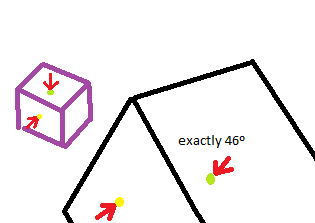(actually i just noticed the green point arrow should exactly on the other side)
The yellow point indicates where the ray hit and the green point where i want to get (i know how to get that position and i only need it for height)
This would be the same part that’s not rotated: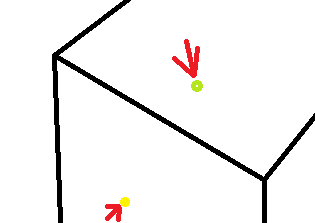But then you’d say That’s simple, if you can do that then why cant you get the rotation data?
Well… heres the problem.
i was searching online on how would i go about getting surface data and i found this function by an user called “adark”. Well im a person that likes to do everything by myself but i’m not the one to reinvent the wheel. As long as i understand what i’m using its okay by me.
this is his code:

``````local hit, position, norm = RayCast(startPos, direction, tool.Parent)

if hit then
wait()
print(norm)
norm = hit.CFrame:vectorToObjectSpace(norm) --This is where the magic happens.
local x,y,z = norm.X, norm.Y, norm.Z
if x ~= 0 and (y == 0 and z == 0) then
hitx = true
if x > 0 then
print("Front")
else
print("Back")
end
elseif y ~= 0 and (x == 0 and z == 0) then
hity = true
if y > 0 then
print("Top")
else
print("Bottom")
end
elseif z ~= 0 and (x == 0 and y == 0) then
hitz = true
if z > 0 then
print("Left") --These two might be backwards!
else
print("Right")
end
end
end
``````

By experimenting a little with it i found out most of the rotations were mesed up so i fixed them. in the end it turned out to be like this:

``````function Get_Surface(hit,pos,norm)
norm = hit.CFrame:vectorToObjectSpace(norm) --This is where the magic happens.
local x,y,z = norm.X, norm.Y, norm.Z
local rot=0
local num=0
local sur=""
if x ~= 0 and (y == 0 and z == 0) then
hitx = true
if x > 0 then
num=0
rot=hit.Rotation.X
sur="Right"
else
num=0
rot=hit.Rotation.X
sur="Left"
end
elseif y ~= 0 and (x == 0 and z == 0) then
hity = true
if y > 0 then
num=1
rot=hit.Rotation.Y
sur="Top"
else
num=1
rot=hit.Rotation.Y
sur="Bottom"
end
elseif z ~= 0 and (x == 0 and y == 0) then
hitz = true
if z > 0 then
num=2
rot=hit.Rotation.Z
sur="Back"
else
num=2
sur="Front"
rot=hit.Rotation.Z
end
end
return {num,rot,sur,x,y,z}
end
``````

and my full script like this:

``````repeat wait() until workspace.DevSersponge
u2=UDim2.new
it=Instance.new
v3=Vector3.new

bbg.Size = u2(2,0,1,0)
bbg.AlwaysOnTop = true
bbg.StudsOffsetWorldSpace = v3(0,3,0)
ttb = Instance.new("TextBox", bbg)
ttb.Size = u2(2, 0, 1, 0)
ttb.Position = u2(-0.5, 0, -0.5, 0)
ttb.BackgroundTransparency = 1
ttb.TextStrokeTransparency=0
ttb.TextColor3=Color3.new(1,1,1)
ttb.TextScaled = true
ttb.Text = " "

function Get_Surface(hit,pos,norm)
norm = hit.CFrame:vectorToObjectSpace(norm) --This is where the magic happens.
local x,y,z = norm.X, norm.Y, norm.Z
local rot=0
local num=0
local sur=""
if x ~= 0 and (y == 0 and z == 0) then
hitx = true
if x > 0 then
num=0
rot=hit.Rotation.X
sur="Right"
else
num=0
rot=hit.Rotation.X
sur="Left"
end
elseif y ~= 0 and (x == 0 and z == 0) then
hity = true
if y > 0 then
num=1
rot=hit.Rotation.Y
sur="Top"
else
num=1
rot=hit.Rotation.Y
sur="Bottom"
end
elseif z ~= 0 and (x == 0 and y == 0) then
hitz = true
if z > 0 then
num=2
rot=hit.Rotation.Z
sur="Back"
else
num=2
sur="Front"
rot=hit.Rotation.Z
end
end
return {num,rot,sur,x,y,z}
end
hrp=workspace.DevSersponge.HumanoidRootPart
grabbing=false
game:GetService("RunService").Heartbeat:Connect(function(dt)
local target=(hrp.CFrame.p+hrp.CFrame.LookVector*10-hrp.CFrame.p).unit*10
local ray = rn(hrp.CFrame.p,target);
local hit, pos, normal = workspace:FindPartOnRayWithIgnoreList(ray, {workspace.DevSersponge,script});

if hit then
if hit.Parent==nil then return end
if hit:FindFirstChildOfClass("Humanoid") then return end
local npos=cf(pos+normal*math.huge)
pcall(function()
local surface=Get_Surface(hit,pos,normal)
ttb.Text=("NAME: "..surface.." ROT: "..surface.." NUM: "..surface.." VEC: "..tostring(Vector3.new(surface,surface,surface)))
end)
end
end)
``````

But this is what happens: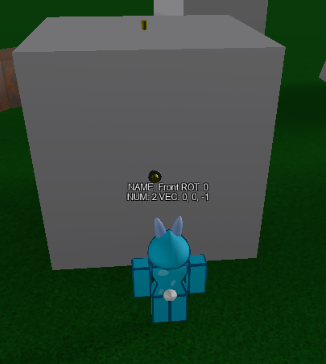–properly gets the surface (not rotated)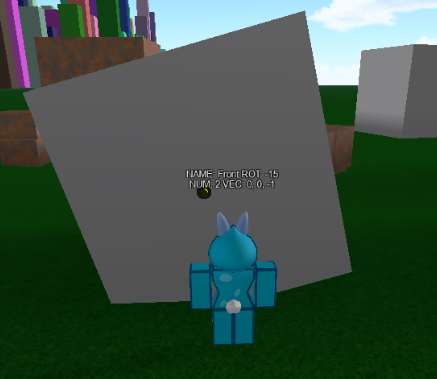–properly gets the surface and rotation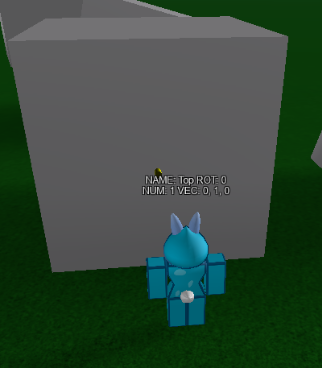–again properly gets the sruface (not rotated)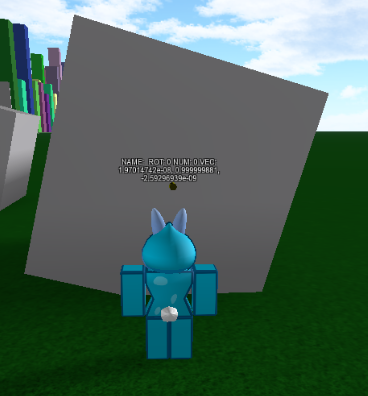–what?..
Literally gets no surface,no rotation data and the Vector is messed up even though its a unit vector.
the vector is defined on this line in case you havent seen

``````norm = hit.CFrame:vectorToObjectSpace(norm)
``````

yes i have tried using math.clamp but 1. it still doesnt work and 2.if i cant get the rotation and surface theres no point

Taking a closer look this is the returned data: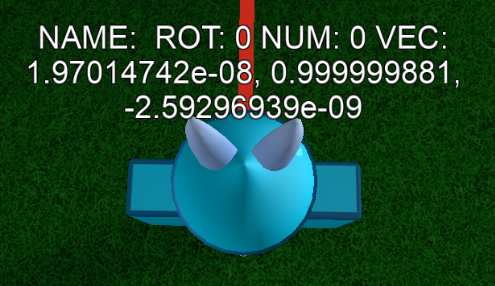Does anyone know why is this happening? what am i doing wrong?

Would be of great help if someone answeredI always wanted to make ledge grabbing when i was a lua beginner and now that i know alot of stuff i have been facing those advanced problemsThank you for reading.

TL;DR:

I want to get the player’s closest top position relative to the player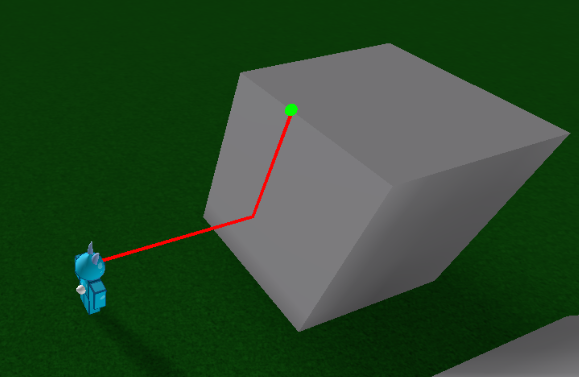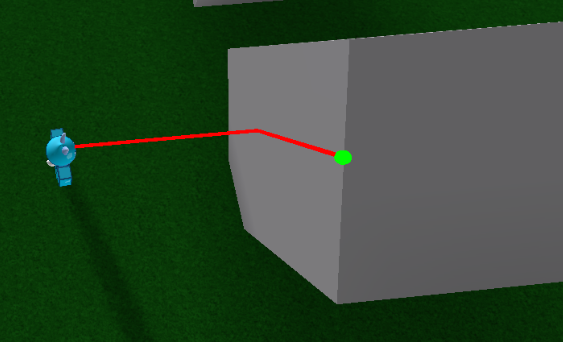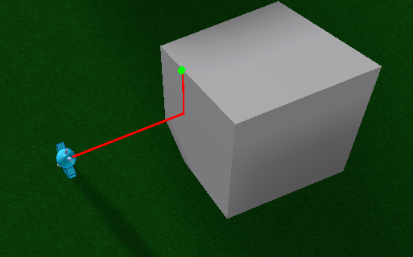4 Likes